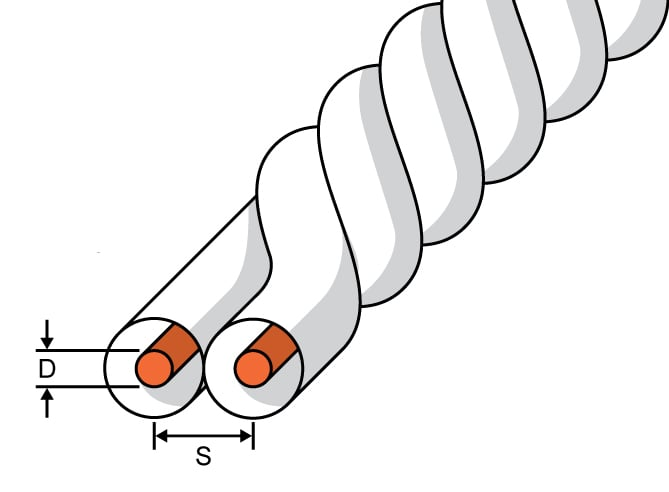# Twisted-Pair Impedance Calculator

## A tool designed to calculate the characteristic impedance of a twisted-pair cable

Ohms
ns/in
nH/in
pF/in

### Overview

A twisted-pair cable is simply two wires that are twisted together so as to reduce radiated EMI (electromagnetic interference) and mitigate the effects of received EMI. Twisted pairs are used with balanced signals. It is important to determine the characteristic impedance of a twisted-pair cable because this impedance should match the impedance of the transmitting and receiving circuitry.

This calculator computes the characteristic impedance of a twisted-pair cable based on its dimensions. This tool will also provide the signal propagation delay, capacitance, and inductance per unit length.### Equations

$$Zo_{twistedpair}=\frac{120}{\sqrt{e_{r}}}\cdot \ln \left [ \frac{2s}{d} \right ]$$

$$delay=84.72\cdot10^{-3}\cdot \sqrt{e_{r}}$$

$$L_{twistedpair} =10.16\cdot 10^{-9}\cdot \ln \left [ \frac{2s}{d} \right ]$$

$$C_{twistedpair} =\left ( \frac{.7065}{\ln \left ( \frac{2s}{d} \right )} \right )\cdot e_{r}$$

### Applications

Twisted-pair cable is especially valuable when used with two signals that transmit information differentially, i.e., using positive and negative versions of the same signal. A differential system can also be referred to as a balanced system, because the two signals are "balanced" on either side of the common-mode voltage. A differential receiver subtracts the negative signal from the positive one, meaning that any voltage components present in both signals will be eliminated. This makes differential systems more robust against noise—as long as the noise is present in both signals. This is why twisted pair is the proper cabling for differential signals: the close physical proximity of the two wires ensures that EMI will be coupled almost equally into both conductors, which in turn ensures that the differential receiver circuitry will eliminate this noise.

Twister pair is also useful when it is important to minimize the generation of EMI. Balanced signals will generate EMI with opposing polarity, such that the EMI is largely canceled out. This EMI-canceling effect is more pronounced when the conductors are in close proximity.

Twisted-pair cable is helpful even without shielding, but a shielded cable will provide further protection against EMI.

As indicated by the above formula, the impedance of a twisted-pair cable is influenced by the spacing and diameter of the conductors.•AEKron April 20, 2016

Whew! That was a lot of bookmarking today!

Like.
• M
mofilippov February 27, 2019

Thank you, it is very useful.
I did not find from the equations how density of twists will affect the pair impedance.

Like.
•Gabor_Sz April 30, 2020

It looks like this is a simplified formula that doesn’t take into count the twisting density.  The image shows a very tightly twisted pair which should mean a lower effective dielectric constant and a slower velocity of propagation.  Typical twisted pairs in multi-pair cable are much more loosely twisted so that the insulation of the two wires in the pair does not touch the insulation of the next twist.  This should more closely match the formula above than the very tightly twisted wire shown in the illustration.

Like.